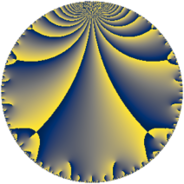# Properties

 Label 90.6.iLevel $90$ Weight $6$ Character orbit 90.i Rep. character $\chi_{90}(49,\cdot)$ Character field $\Q(\zeta_{6})$ Dimension $60$ Newform subspaces $1$ Sturm bound $108$ Trace bound $0$

# Learn more about

## Defining parameters

 Level: $$N$$ $$=$$ $$90 = 2 \cdot 3^{2} \cdot 5$$ Weight: $$k$$ $$=$$ $$6$$ Character orbit: $$[\chi]$$ $$=$$ 90.i (of order $$6$$ and degree $$2$$) Character conductor: $$\operatorname{cond}(\chi)$$ $$=$$ $$45$$ Character field: $$\Q(\zeta_{6})$$ Newform subspaces: $$1$$ Sturm bound: $$108$$ Trace bound: $$0$$

## Dimensions

The following table gives the dimensions of various subspaces of $$M_{6}(90, [\chi])$$.

Total New Old
Modular forms 188 60 128
Cusp forms 172 60 112
Eisenstein series 16 0 16

## Trace form

 $$60q + 480q^{4} + 58q^{5} - 80q^{6} - 754q^{9} + O(q^{10})$$ $$60q + 480q^{4} + 58q^{5} - 80q^{6} - 754q^{9} + 104q^{11} - 1528q^{14} - 2780q^{15} - 7680q^{16} - 928q^{20} + 11776q^{21} - 640q^{24} + 3306q^{25} - 16224q^{26} - 5262q^{29} - 3128q^{30} + 8868q^{31} + 5072q^{35} - 13568q^{36} - 9708q^{39} + 41546q^{41} + 3328q^{44} + 61748q^{45} - 18960q^{46} + 70200q^{49} - 19376q^{50} - 45008q^{51} - 52696q^{54} - 984q^{55} + 24448q^{56} - 34228q^{59} - 59776q^{60} - 23370q^{61} - 245760q^{64} + 77184q^{65} - 65104q^{66} + 148898q^{69} - 26664q^{70} + 96744q^{71} - 99760q^{74} + 57716q^{75} + 17448q^{79} - 29696q^{80} + 335146q^{81} + 132128q^{84} - 40812q^{85} - 75440q^{86} + 1011388q^{89} + 169888q^{90} + 64680q^{91} + 48120q^{94} + 376884q^{95} + 10240q^{96} - 1412012q^{99} + O(q^{100})$$

## Decomposition of $$S_{6}^{\mathrm{new}}(90, [\chi])$$ into newform subspaces

Label Dim. $$A$$ Field CM Traces $q$-expansion
$$a_2$$ $$a_3$$ $$a_5$$ $$a_7$$
90.6.i.a $$60$$ $$14.435$$ None $$0$$ $$0$$ $$58$$ $$0$$

## Decomposition of $$S_{6}^{\mathrm{old}}(90, [\chi])$$ into lower level spaces

$$S_{6}^{\mathrm{old}}(90, [\chi]) \cong$$ $$S_{6}^{\mathrm{new}}(45, [\chi])$$$$^{\oplus 2}$$